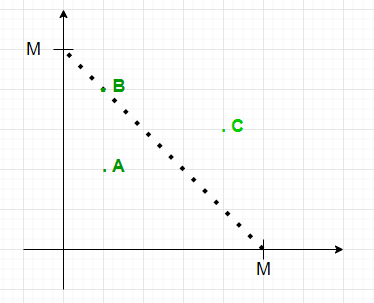Queries to count points lying on or inside an isosceles Triangle with given length of equal sides

• Last Updated : 01 Jul, 2021

Given an array arr[][] of dimension N * 2 representing the co-ordinates of N points and an array Q[] consisting of M integers, the task for every element in the Q[i] is to find the number of points lying inside or on the right-angled isosceles triangle formed on the positive co-ordinate axis with two equal sides of length Q[i]in each query.

Examples:

Input: N = 4, arr[][] = { {2.1, 3.0}, {3.7, 1.2}, {1.5, 6.5}, {1.2, 0.0} }, Q[] = { 2, 8, 5}, M = 3
Output: 1 4 2
Explanation:

• First query: The point (1.2, 0.0) lies inside the triangle.
• Second query: The points { (2.1, 3.0), (3.7, 1.2), (1.2, 0.0) } lies inside the triangle and point (1.5, 6.5) lies on the triangle.
• Third query: The points {(3.7, 1.2), (1.2, 0.0)} lies inside the triangle.

Input: N = 3, arr[][] = { {0, 0}, {1, 1}, {2, 1} }, Q[] = {1, 2, 3}, M = 3
Output: 1 2 3
Explanation:

• First query: The point (0, 0) lies inside the triangle.
• Second query: The points { (0, 0), (1, 1) } lies inside the triangle.
• Third query: All the points lies inside the triangle.

Naive Approach: The simplest approach is to in each query traverse the array of points and checks if it lies inside the right-angled triangle formed. After completing the above steps, print the count.
Time Complexity: O(N * M)
Auxiliary Space: O(1)

Efficient Approach: The above approach can be optimized based on the following observations:• A point (x, y) lies inside the isosceles right angle triangle formed on co-ordinate axis whose two equal sides are X if :
• x ≥ 0 && y ≥ 0 && x + y ≤ X
• By pre-storing the count of points with coordinates sum ≤ X, the query can be answered in constant time.

Follow the steps below to solve the problem:

Below is the implementation of the above approach:

C++

 // C++ implementation of above approach #include using namespace std; int const MAX = 1e6 + 5; // Function to find answer of each queryint query(vector > arr,          vector Q){    // Stores the count of points with sum    // less than or equal to their indices    int pre[MAX] = { 0 };     // Traverse the array    for (int i = 0; i < arr.size(); i++) {         // If both x and y-coordinate < 0        if (arr[i] < 0 || arr[i] < 0)            continue;         // Stores the sum of co-ordinates        int sum = ceil((arr[i] + arr[i]));         // Increment count of sum by 1        pre[sum]++;    }     // Prefix array    for (int i = 1; i < MAX; i++)        pre[i] += pre[i - 1];     // Perform queries    for (int i = 0; i < Q.size(); i++) {        cout << pre[Q[i]] << " ";    }    cout << endl;}// Drivers Codeint main(){    vector > arr = { { 2.1, 3.0 },                                   { 3.7, 1.2 },                                   { 1.5, 6.5 },                                   { 1.2, 0.0 } };    vector Q = { 2, 8, 5 };    int N = arr.size();    int M = Q.size();     query(arr, Q);}

Java

 // Java implementation of above approachimport java.util.*;class GFG{ static int MAX = (int) (1e6 + 5); // Function to find answer of each querystatic void query(double [][]arr,          int []Q){       // Stores the count of points with sum    // less than or equal to their indices    int pre[] = new int[MAX];     // Traverse the array    for (int i = 0; i < arr.length; i++)    {         // If both x and y-coordinate < 0        if (arr[i] < 0 || arr[i] < 0)            continue;         // Stores the sum of co-ordinates        int sum = (int) Math.ceil((arr[i] + arr[i]));         // Increment count of sum by 1        pre[sum]++;    }     // Prefix array    for (int i = 1; i < MAX; i++)        pre[i] += pre[i - 1];     // Perform queries    for (int i = 0; i < Q.length; i++)    {        System.out.print(pre[Q[i]]+ " ");    }    System.out.println();}   // Drivers Codepublic static void main(String[] args){    double[][] arr = { { 2.1, 3.0 },                                   { 3.7, 1.2 },                                   { 1.5, 6.5 },                                   { 1.2, 0.0 } };    int []Q = { 2, 8, 5 };    int N = arr.length;    int M = Q.length;     query(arr, Q);}} // This code is contributed by 29AjayKumar

Python3

 # Python3 implementation of above approachMAX = 10**6 + 5from math import ceil # Function to find answer of each querydef query(arr, Q):       # Stores the count of points with sum    # less than or equal to their indices    pre = *(MAX)     # Traverse the array    for i in range(len(arr)):         #` If both x and y-coordinate < 0        if (arr[i] < 0 or arr[i] < 0):            continue         # Stores the sum of co-ordinates        sum = ceil((arr[i] + arr[i]));         # Increment count of sum by 1        pre[sum] += 1     # Prefix array    for i in range(1, MAX):        pre[i] += pre[i - 1]     # Perform queries    for i in range(len(Q)):        print(pre[Q[i]], end = " ")         # Drivers Codeif __name__ == '__main__':    arr = [[ 2.1, 3.0],          [ 3.7, 1.2],          [ 1.5, 6.5],          [ 1.2, 0.0]]    Q = [2, 8, 5]    N = len(arr)    M = len(Q)     query(arr, Q)     # This code is contributed by mohit kumar 29.

C#

 // C# implementation of above approachusing System;public class GFG{   static int MAX = (int) (1e6 + 5);   // Function to find answer of each query  static void query(double [,]arr,                    int []Q)  {     // Stores the count of points with sum    // less than or equal to their indices    int []pre = new int[MAX];     // Traverse the array    for (int i = 0; i < arr.GetLength(0); i++)    {       // If both x and y-coordinate < 0      if (arr[i,0] < 0 || arr[i,1] < 0)        continue;       // Stores the sum of co-ordinates      int sum = (int) Math.Ceiling((arr[i,0] + arr[i,1]));       // Increment count of sum by 1      pre[sum]++;    }     // Prefix array    for (int i = 1; i < MAX; i++)      pre[i] += pre[i - 1];     // Perform queries    for (int i = 0; i < Q.Length; i++)    {      Console.Write(pre[Q[i]]+ " ");    }    Console.WriteLine();  }   // Drivers Code  public static void Main(String[] args)  {    double[,] arr = { { 2.1, 3.0 },                     { 3.7, 1.2 },                     { 1.5, 6.5 },                     { 1.2, 0.0 } };    int []Q = { 2, 8, 5 };    int N = arr.GetLength(0);    int M = Q.Length;    query(arr, Q);  }} // This code is contributed by 29AjayKumar

Javascript



Output:
1 4 2

Time Complexity: O(N)
Auxiliary Space: O(N)

My Personal Notes arrow_drop_up